#INFOSWMM

# Surface Runoff in InfoSWMM and InfoSWMM SA

InfoSWMM and InfoSWMM SA offer 13 choices for modeling surface runoff (click on link to see more information on each method):#### Nonlinear Reservoir

The conceptual view of surface runoff used by InfoSWMM H2OMap SWMM InfoSWMM SA is illustrated in the figure below. Each Subcatchment surface is treated as a nonlinear reservoir. Inflow comes from precipitation and upstream catchments. There are several outflows, including infiltration, evaporation, and surface runoff. The capacity of this "reservoir" is the maximum depression storage, which is the maximum surface storage provided by ponding, surface wetting, and interception. Surface runoff per unit area, Q, occurs only when the depth of water in the "reservoir" exceeds the maximum depression storage, dp, in which case the outflow is given by Manning's equation.Depth of water over the Subcatchment (d in feet) is continuously updated with time (t in seconds) by solving numerically a water balance equation over the Subcatchment.

Infiltration data for CN infiltration, Horton or Green-Ampt infiltration on the Subcatchments are only used if the runoff option for a Nonlinear reservoir is used. If you are using the NRSCS Unit Hydrograph method the losses are computed from the Subcatchment CN and NOT the infiltration CN.#### The Colorado Urban Hydrograph Procedure (CUHP)

The Colorado Urban Hydrograph Procedure (CUHP) uses the equations and procedures presented in the Urban Drainage Criteria Manual (USDCM) of the Urban Drainage and Flood Control District (UDFCD 2001). Shape of the CUHP synthetic unit hydrograph is determined using the following equations that relate unit hydrograph parameters to catchment properties.

The Colorado Urban Hydrograph Procedure (CUHP) uses the equations and procedures presented in the Urban Drainage Criteria Manual (USDCM) of the Urban Drainage and Flood Control District (UDFCD 2001). Shape of the CUHP synthetic unit hydrograph is determined using the following equations that relate unit hydrograph parameters to catchment properties.

Lag time (tl) of the watershed (catchment), defined as the time from the center of unit storm duration to the peak of the unit hydrograph, is determined as: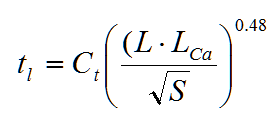where tl = lag time in hours.

L = length along the drainageway path from study point to the most upstream limits of

the catchment in miles.

Lca = length along stream from study point to a point along stream adjacent to the centroid

of the catchment in miles.

S = length weighted average slope of catchment along drainageway path to upstream

limits of the catchment.

Ct = time to peak coefficient.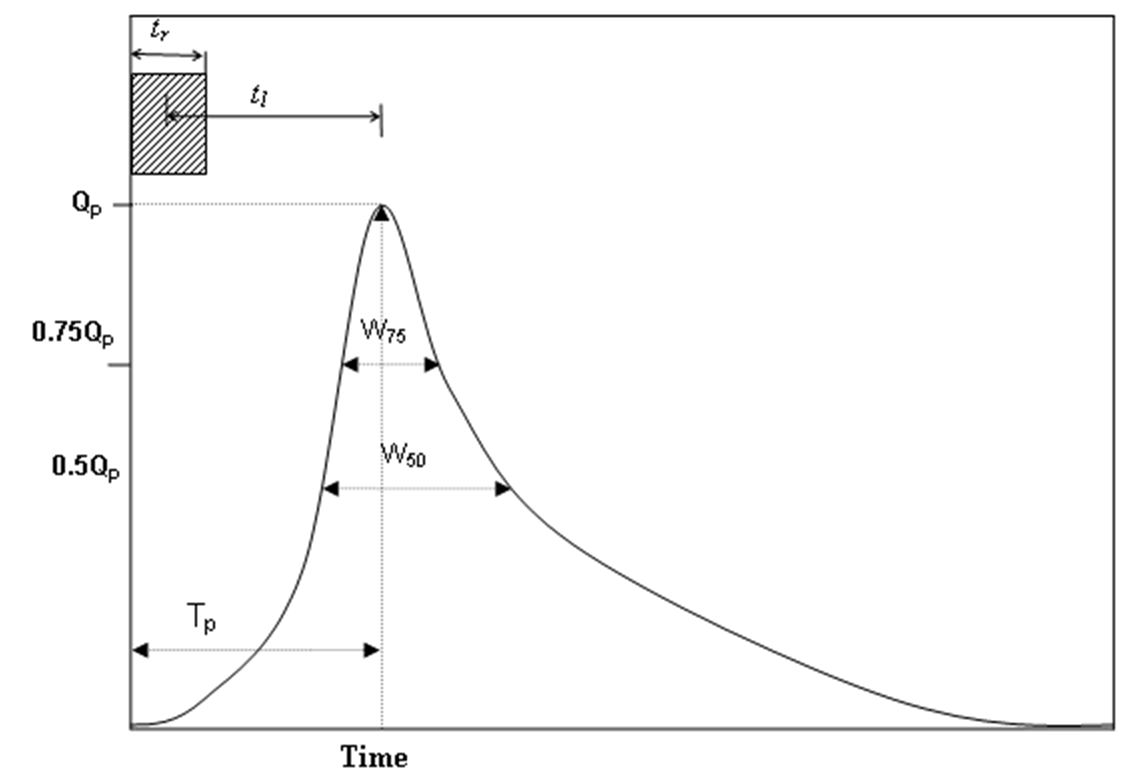Once the lag time is known, time to peak (Tp) of the unit hydrograph could be determined by adding 0.5tr to the lag time in consistent units.

Peak flow rate, Qp, of the unit hydrograph is calculated as:where Qp = peak flow rate of the unit hydrograph, in cfs.

A = area of the catchment, in square miles.

Cp = unit hydrograph peaking coefficient, and is determined as: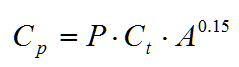where P = peaking parameter.

Ct and P are defined in terms of percent impervious (Ia) of the catchment as:The coefficients a, b, c, d, e, and f are defined in terms of Ia in the following table.

 IA A B C D E F Ia ≤ 10 0.0 -0.00371 0.163 0.00245 -0.012 2.16 10 < Ia ≤ 40 0.000023 -0.00224 0.146 0.00245 -0.012 2.16 Ia > 40 0.0000033 -0.000801 0.120 -0.00091 0.228 -2.06

The widths of the unit hydrograph at 50% and 75% of the peak are estimated as: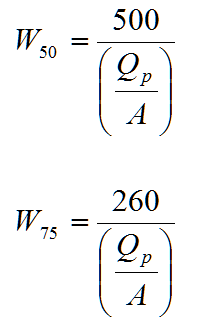where W50 = width of the unit hydrograph at 50% of the peak, in hours.

W75 = width of the unit hydrograph at 75% of the peak, in hours.

Qp = peak flow rate, in cfs.

A = catchment area, in square miles.

It is recommended that a unit hydrograph duration of 5-minute be used for studies that apply the CUHP. The maximum recommended drainage area (catchment size) for any single CUHP unit hydrograph is 5 square miles. Whenever a larger watershed is studied, it needs to be subdivided into Subcatchments of 5-square miles or less. For this synthetic unit hydrograph method, the minimum drainage area should be 90 acres. For catchments smaller than 90 acres, other unit hydrograph generation mechanisms should be used.

#### NRCS (SCS) Dimensionless Unit Hydrograph Method

A unit hydrograph is defined as the direct runoff hydrograph resulting from a unit depth of excess (effective) rainfall produced by a storm of uniform intensity and specified duration. Unit hydrographs could be natural or synthetic. Natural unit hydrographs are derived from observed data, whereas synthetic unit hydrographs are generated following empirical techniques based on watershed parameters and storm characteristics to simulate the natural unit hydrograph. In addition to the rational formula, H2OCalc computes peak flow from a watershed using the NRCS (SCS) dimensionless unit hydrograph.

The NRCS dimensionless unit hydrograph, graphically descried below, is widely used in practice. To generate a tr-hour unit hydrograph for a watershed, time to peak (Tp) and the peak flow rate (Qp) are determined using watershed characteristics.where tr is duration of effective rainfall, and tl is lag time of the watershed. Lag time represents the time from the center of mass of effective rainfall to the time to peak of a unit hydrograph. In other words, lag time is a delay in time, after a brief rain over a watershed, before the runoff reaches its peak. The lag time can either be specified by the user, or can be calculated by the model using the following SCS equation.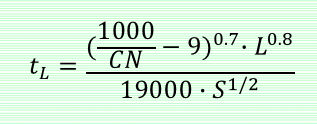where tl = lag time of the watershed (hr).

L = hydraulic length of the watershed (ft). This refers to travel distance of water from the most upstream location of the watershed to the point where the unit hydrograph is derived.

CN = the SCS curve number. This is a measure of runoff generating capacity of a watershed, and it depends on the soil, the antecedent moisture condition, the cover, and the hydrologic conditions of the watershed. Recommended CN values are given in the following table for urban areas (USDA 1986). The SCS suggests the CN values for the above equation to be within 50 and 95.

S = average slope of the watershed.

The peak flow rate is calculated as:where Qp = peak flow rate (ft3/s).

A = area of the watershed, in square miles, draining to the location of the unit hydrograph.

Tp = time to peak of the unit hydrograph in hours.

Once Tp and Qp are known, actual time and flow rate ordinates of the tr-hour unit hydrograph are determined by multiplying the dimensionless time (T/Tp) and the dimensionless flow rate ordinates (Q/Qp) by Tp and Qp, respectively.NRCS Dimensionless Unit Hydrograph

Storm hydrograph could be computed using the unit hydrograph and a given precipitation pattern. The precipitation could be a design precipitation or actual (i.e., historical precipitation). A design precipitation may be specified in the form of rainfall depth over 24-hr duration. Distribution of the rainfall depth across the 24-hour can be estimated using the SCS (NRCS) rainfall types (i.e. Type I, Type IA, Type II and Type III) shown in the following figure.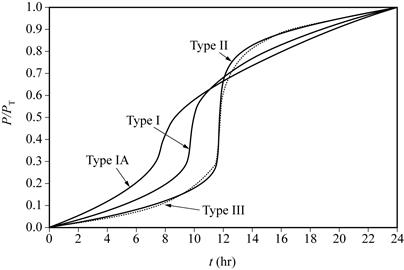SCS (NRCS) Rainfall Types (Source Nicklow et al., 2006)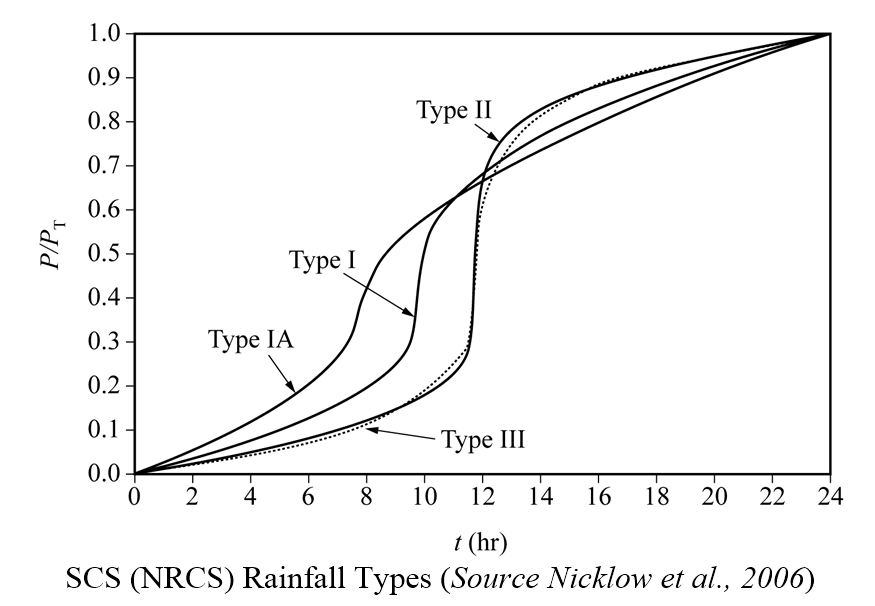Application Notes:

·

• If the Time of Concentration (Tc) field is left blank (null) then the Tc will be computed internally in minutes as Tc = L0.8[(1000/CN)-9]0.7/(19S0.5), where L is Length in feet and S is Subcatchment Slope in %. This value is reported in the Simulation Report (see below).
• If infiltration and soil coverage data is defined for a Subcatchment, then a composite Curve Number will be computed and used for rainfall infiltration calculations. This value is reported in the Simulation Report (see below). You can use this composite Curve Number for routing purposes as well if you desire by copying the value to the Curve Number field in the Attribute Browser for each Subcatchment.
• The Depression Storage field is used as Initial Abstraction for this method (i.e. no runoff will occur until the cumulative rainfall is greater than Depression Storage).#### NRCS (SCS) Triangular Unit Hydrograph Method

The SCS has also developed a triangular unit hydrograph (USDA 1986) (see figure below) that is an approximation to the dimensionless unit hydrograph described above. The triangular unit hydrograph is entirely defined in terms of three points, Qp, Tp, and Tb. The lag time, time to peak, and peak flow rate are calculated using the same equations as for the dimensionless unit hydrograph.

.

#### Delmarva Unit Hydrograph

As previously described, the NRCS dimensionless unit hydrograph and the NRCS triangular unit hydrograph are extensively used to develop storm Hydrographs for hydrologic evaluation and design of soil and water resources management practices throughout the United States. However, in areas such as the Delmarva Peninsula where the local topography is flat and where considerable surface storage is available, shape of observed storm Hydrographs significantly differ from those generated using the NRCS unit Hydrographs. As a result, a unit hydrograph that is similar with the NRCS dimensionless unit hydrograph, but with modifications to better represent the runoff characteristics of the Delmarva Peninsula has been used by utilities in the states of Delaware, Maryland, Virginia, and some parts of New Jersey. This unit hydrograph is known as the Delmarva unit hydrograph.

The Delmarva unit hydrograph uses the following equation to estimate peak flow rate.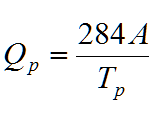where

Qp = peak flow rate in cfs.

A = area of the watershed, in square miles, draining to the location of the unit hydrograph.

Tp = time to peak of the unit hydrograph in hour

Time to peak, and lag time are calculated according to Equations 97 and 98, respectively. When compared with the NRCS methods, the Delmarva unit hydrograph produces lower peak flow rate but yields the same flow volume.

#### Snyder Unit Hydrograph Method

Snyder’s method for unit hydrograph synthesis relates the time from the centroid of the excess rainfall to the peak of the unit hydrograph, also referred to as lag time, to geometric characteristics of the basin in order to derive critical points for interpolating the unit hydrograph. Lag time is evaluated bywhere tL is in hrs; C1 is a constant equal to 1.0 in U.S. customary units and 0.75 in S.I. units; Ct is an empirical watershed storage coefficient, which generally ranges from 1.8 to 2.2; Lis the length of the main stream channel in mi or km; and LCA is the length of stream channel from a point nearest the center of the basin to the outlet in mi or km.

The standard duration of excess rainfall is computed empirically by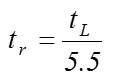Adjusted values of lag time, tLa, for other durations of rainfall excess can be obtained bywhere tra is the alternative unit hydrograph duration. Time to peak discharge can be computed as a function of lag time and duration of excess rainfall, expressed asThe peak discharge, Qp is defined as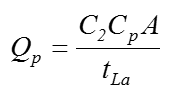where Qp is in cfs/in or m3/s/m; C2 is a constant equal to 640 in U.S. customary units and 2.75 in S.I. units; A is drainage area in mi2 or km2; and Cp is a second empirical constant ranging from approximately 0.5 to 0.7. Coefficients Ct and Cp are regional parameters that should be calibrated or be based on values obtained for similar gaged drainage areas. The ultimate shape of Snyder’s unit hydrograph is primarily controlled by two parameters, W50 and W75, which represents widths of the unit hydrograph at discharges equal to 50 and 75 percent of the peak discharge, respectively. These shape parameters can be evaluated by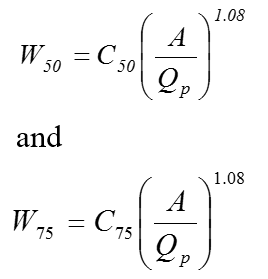where C50 is a constant equal to 770 in U.S.

where C50 is a constant equal to 770 in U.S. customary units and 2.14 in S.I. units; and C75 is a constant equal to 440 in U.S. customary units and 1.22 in S.I. units. The location of the end points for W50 and W75 are often placed such that one-third of both values occur prior to the time to peak discharge and the remaining two-thirds occur after the time to peak. Finally, the base time, or time from beginning to end of direct runoff, should be evaluated such that the unit hydrograph represents 1 in (or 1 cm in S.I. units) of direct runoff volume. With known values of tp, Qp, W50, and W75, along with the adjusted base time, one can then locate a total of seven unit hydrograph ordinates.

#### Clark Unit Hydrograph Method

Clark’s method derives a unit hydrograph by explicitly representing the processes of translation and attenuation, which are the two critical phenomena in transformation of excess rainfall to runoff hydrograph. Translation refers to the movement, without storage, of runoff from its origin to the watershed outlet in response to gravity force, where as attenuation represents the reduction of runoff magnitude due to resistances arising from frictional forces and storage effects of soil, channel, and land surfaces. Clark (1945) noted that the translation of flow through the watershed could be described by a time-area curve (see Figure below), which expresses the curve of the fraction of watershed area contributing runoff to the watershed outlet as a function of travel time since the start of effective precipitation. Each subarea is delineated so that all the precipitation falling on the subarea instantaneously has the same time of travel to the outflow point.

Clark’s unit hydrograph is obtained by routing a unit depth of direct runoff to the channel in proportion to the time-area curve and routing the runoff entering the channel through a linear reservoir. In practical applications, it is usually necessary to alter the IUH into a unit hydrograph of specific duration. This can be accomplished by lagging the IUH by the desired duration and averaging the ordinates. Clark’s method derives a unit hydrograph by explicitly representing the processes of translation and attenuation, which are the two critical phenomena in transformation of excess rainfall to runoff hydrograph. Translation refers to the movement, without storage, of runoff from its origin to the watershed outlet in response to gravity force, where as attenuation represents the reduction of runoff magnitude due to resistances arising from frictional forces and storage effects of soil, channel, and land surfaces. Clark (1945) noted that the translation of flow through the watershed could be described by a time-area curve (see Figure below), which expresses the curve of the fraction of watershed area contributing runoff to the watershed outlet as a function of travel time since the start of effective precipitation. Each subarea is delineated so that all the precipitation falling on the subarea instantaneously has the same time of travel to the outflow point.

Developing a time-area curve for a watershed could be a time consuming process. For watersheds that lack derived time-area diagram, the HEC-HMS model, which was developed at the Hydrologic Engineering Center (HEC) of the U.S. Army Corps of Engineers, uses the following relationship (HEC, 2000)where Ac,t is cumulative watershed area contributing at time t; AT is total watershed area; and tc is time of concentration of the watershed. If the incremental areas, denoted as Ai in the figure below, are multiplied by a unit depth of excess rainfall and divided by Δt, the computational time step, the result is a translated hydrograph that is considered as an inflow to a conceptual linear reservoir located at the watershed outlet.

To account for storage effects, the attenuation process is modeled by routing the translated hydrograph through a linear reservoir with storage properties similar to those of the watershed. The routing model is based on the mass balance equationwhere dS/dt is time rate of change of water in storage at time t; It is average inflow, obtained from the time-area curve, to storage at time t; and Qt is outflow from storage at time t.For linear reservoir model, storage is related to outflow as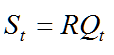where R is a constant linear reservoir parameter that represents the storage effect of the watershed. Usually, lag time (tL) is used as an approximation to R. Combining and solving Equations 116 and 117 using a finite difference approximation provideswhere C1 and C2 are routing coefficients calculated asThe average outflow during period t is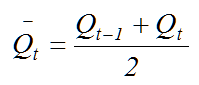If the inflow, It, ordinates are runoff from a unit depth of excess rainfall, the average outflows derived by Equation 121 represent Clark’s unit hydrograph ordinates. Clark’s unit hydrograph is, therefore, obtained by routing a unit depth of direct runoff to the channel in proportion to the time-area curve and routing the runoff entering the channel through a linear reservoir. Note that solution of Equations 118 and 121 is a recursive process. As such, average outflow ordinates of the unit hydrograph will theoretically continue for an infinite duration. Therefore, it is customary to truncate the recession limb of the unit hydrograph where the outflow volume exceeds 0.995 inches or mm. Clark’s method is based on the premise that duration of the rainfall excess is infinitesimally small. Because of this, Clark’s unit hydrograph is referred to as an instantaneous unit hydrograph or IUH. In practical applications, it is usually necessary to alter the IUH into a unit hydrograph of specific duration. This can be accomplished by lagging the IUH by the desired duration and averaging the ordinates.

#### Espey Unit Hydrograph Method

After analyzing runoff records from 41 urban watersheds located in eight different states in the United States with areas ranging from 9 acres to 15 mi2 and with percent imperviousness ranging from 2 to 100, Espey and Altman (1978) developed a 10-minute unit hydrograph for urban watersheds. This unit hydrograph is commonly named as Espey 10-minute unit hydrograph, and the following equations are used to determine its ordinates.According to Espey and Altman (1978) method, slope is calculated as:where tp = time to peak in minutes.

Qp = peak discharge in ft3/sec.

tb = total hydrograph base in minutes.

W50 = hydrograph widths at 50% of the peak discharge rates in minutes.

W75 = hydrograph widths at 75% of the peak discharge rates in minutes.

A = area in mi2.

L = length in ft.

S = watershed slope in ft/f.

IMP = roughness and percent imperviousness.

H = is the elevation difference in the channel bottom at design point and

0.8L upstream (ft).

f = conveyance factor, dimensionless. This factor depends on the percent imperviousness and weighted main channel Manning roughness coefficient is determined graphically from the following figure.The shape parameters, W50 and W75, are to be drawn parallel to the abscissa and are usually located such that one third of the width is located to the left of the time to peak. Once the equations are solved and the seven ordinates that govern the general shape of the 10-minute unit hydrograph are determined, a smooth curve is then fit through these points and adjusted until the volume of direct runoff is equivalent to one inch over the watershed.

#### Santa Barbara Urban Hydrograph Method

As the name implies, this method was first developed for the Santa Barbara County Flood Control and Water Conservation District in 1975. However, its usage is not limited to Santa Barbara; it can be used at other places as well. The method is well suited for urban applications since it explicitly models impervious areas and pervious areas in a watershed.

The Santa Barbara Urban Hydrograph method generates the actual runoff hydrograph, not a unit hydrograph. According to this method losses from rainfall such as infiltration losses are neglected for the impervious areas, and are considered for pervious areas. Horton’s method is used to determine infiltration losses from pervious areas for the method implemented in InfoSWMM . Once effective rainfall (i.e. rainfall excess) from the impervious and pervious portions f the watershed is known, an instantaneous hydrograph is determined. As the name implies, this method was first developed for the Santa Barbara County Flood Control and Water Conservation District in 1975. However, its usage is not limited to Santa Barbara; it can be used at other places as well. The method is well suited for urban applications since it explicitly models impervious areas and pervious areas in a watershed. Once effective rainfall (i.e. rainfall excess) from the impervious and pervious portions of the watershed is known, an instantaneous hydrograph is determined according to the following equation:One can see from the above equation that the instantaneous hydrograph is calculated based on the assumption that the rainfall excess is produced at the outlet of the subbasin. To account for the time actually needed to transport the effective rainfall to the subbasin outlet, the instantaneous hydrograph is routed through an imaginary linear reservoir according to the following equations to cause a time delay equal to the time of concentration of the subbasin.#### San Diego Modified Rational Formula

The Rational method is a widely used technique for estimation of peak flows from urban and rural drainage basins (Maidment 1993; Mays 2001).

Stormwater modeling applications such as the design of detention basins require knowledge of total inflow volume obtained from runoff hydrographs. For these applications peak flow information alone may not be sufficient. The San Diego modified rational formula is a technique adopted by the San Diego County to generate runoff hydrograph by extending the traditional rational formula.

The San Diego County uses a 6-hour storm event for many stormwater design applications. Accordingly, for designs that are dependent on total storm volume a hydrograph has to be generated by creating a rainfall distribution consisting of blocks of rain, creating an incremental hydrograph for each block of rain using the modified rational formula, and adding the hydrographs from each block of rain where each rainfall block lasts for a duration of time of concentration. The rainfall blocks are distributed across the 6-hour duration using the “2/3, 1/3” distribution in which the peak rainfall block is centered at the 4-hour time within the 6-hour rainfall duration, and the additional blocks are distributed in a sequence alternating two blocks to the left and one block to the right of the 4-hour time.The Rational method is a widely used technique for estimation of peak flows from urban and rural drainage basins (Maidment 1993; Mays 2001). Mathematically, the Rational formula is expressed as: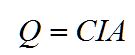where Q = peak runoff rate (flow unit)

C = runoff coefficient (unitless)

I = rainfall intensity (intensity unit)

A = watershed area (area unit)

Stormwater modeling applications such as the design of detention basins require knowledge of total inflow volume obtained from runoff Hydrographs. For these applications peak flow information alone may not be sufficient. The San Diego modified rational formula is a technique adopted by the San Diego County to generate runoff hydrograph by extending the traditional rational formula.

The San Diego County uses a 6-hour storm event for many Stormwater design applications. Accordingly, for designs that are dependent on total storm volume a hydrograph has to be generated by creating a rainfall distribution consisting of blocks of rain, creating an incremental hydrograph for each block of rain using the modified rational formula, and adding the Hydrographs from each block of rain where each rainfall block lasts for a duration of time of concentration. The rainfall blocks are distributed across the 6-hour duration using the “2/3, 1/3” distribution in which the peak rainfall block is centered at the 4-hour time within the 6-hour rainfall duration, and the additional blocks are distributed in a sequence alternating two blocks to the left and one block to the right of the 4-hour time. The actual rainfall amount for each rainfall block is calculated as: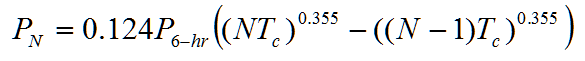where PN = actual rainfall amount for each rainfall block (inch or mm)

P6-hr = six-hour storm depth (inch or mm)

N = an integer representing position of a given block number of rainfall. N

is 1 for the peak rainfall block, and is assigned according to the “2/3,

1/3” distribution rule for other rainfall blocks.

Tc = time of concentration (minutes)

Once rainfall distribution is created, triangular hydrograph is generated for each rainfall block. Peak flow for the triangular hydrograph is computed according to the rational formula. Finally, the overall hydrograph for the 6-hr storm event is determined by adding all the triangular Hydrographs from each block of rain. The final hydrograph has its peak at 4 hours plus ½ of the Tc. The total volume under the hydrograph is the product of runoff coefficient, the six-hour precipitation depth, and area of the Subcatchment.

#### Modified Rational Method

The Rational method is a widely used technique for estimation of peak flows from urban and rural drainage basins (Maidment 1993; Mays 2001). Stormwater modeling applications such as the design of detention basins require knowledge of total inflow volume obtained from runoff hydrographs. For these applications peak flow information alone may not be sufficient. The San Diego modified rational formula is a technique adopted by the San Diego County to generate runoff hydrograph by extending the traditional rational formula. However, the San Diego Modified Rational Method (MRM) has an alternating balance hyetograph and not a constant rainfall. Starting with the release of InfoSWMM and H2Omap SWMM v13 Update 9 a new Modified Rational Method has been added with a constant Rainfall.

#### German Runoff

The source of the equations for the German Runoff hydrology method Is the program Storm XXL which can be seen at http://www.sieker.de/daten/download/sieker/public/manual_storm2006.pdf

It is a linear storage equation and has two parameters (a Storage Constant in minutes) and a reservoir count that is integer and normally ranges from 1 to 5.

Paulsen (1987) gives this equation as a solution for the differential equation system for dt <= K. The effect of the model parameters n and K are similar. A large value for the storage constant K enlarges the retention period and curbs the runoff hydrology. A large number for n also has a dampening effect.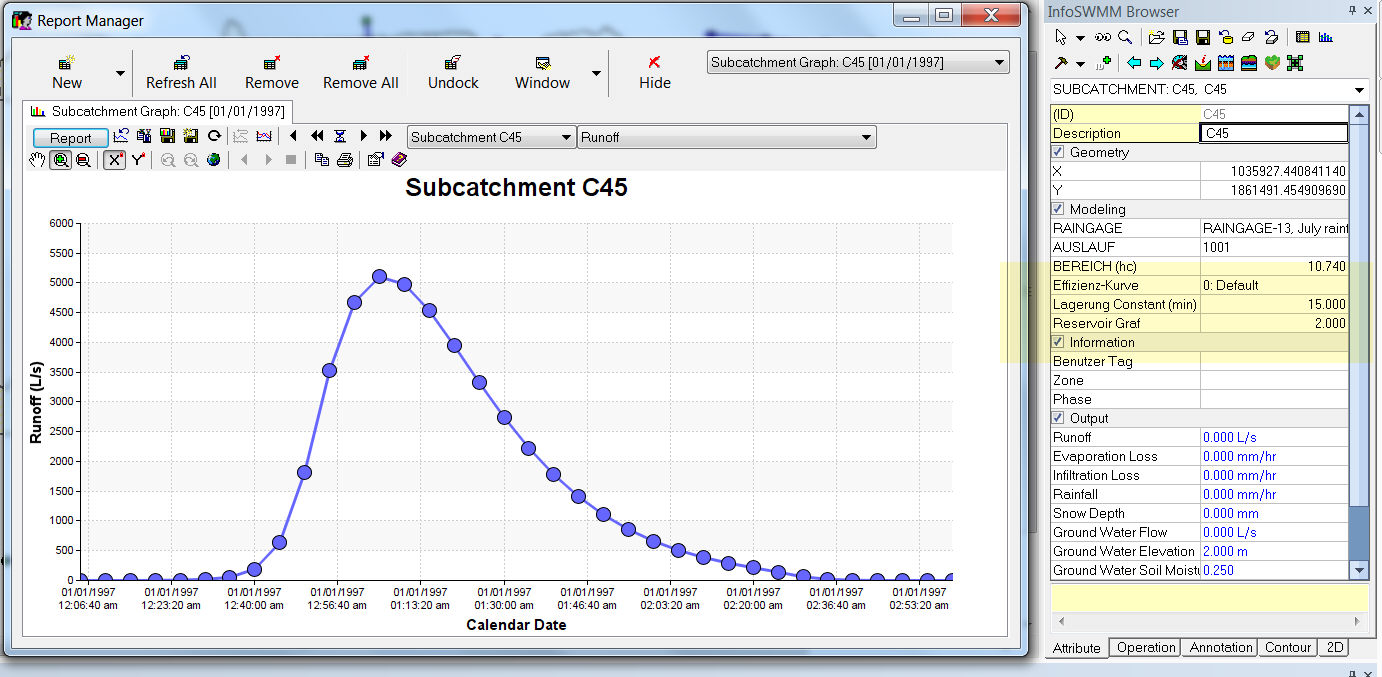#### Rules and Ranges the for Hydrology Options

 Innovyze Hydrology Type Data Ranges and Default Hydrology Type Data Type User Unit Internal Unit Valid Internal Range Default Internal Values rain-depth Colorado UH in or mm DCIA level enumerator 1, 2, 3 1 hydraulic length double ft or m ft >= 1 50 centroid distance double ft or m ft >= 0 0 DCIA fraction double 0 <= & <= 1 0.2 RPA fraction double 0 <= & <= 1 0.75 time of concentration double minutes seconds >= 1 60 captured volume double rain-depth ft >= 0 0 Drainage time double hours seconds >= 0 12 NRCS/Delmarva Curve Number double 40 <= & <= 100 75 hydraulic length double ft or m ft >= 1 50 time of concentration double minutes seconds >= 1 60 depression storage double rain-depth ft >= 0 0 Snyder UH storage coefficient double 0.1 <= & <= 8 2 empirical coefficient double 0.1 <= & <= 1.0 0.5 hydraulic length double ft or m ft >= 1 50 centroid distance double ft or m ft >= 0 0 depression storage double rain-depth ft >= 0 0 Clark UH storage coefficient double 0.1 <= & <= 10 1 time of concentration double minutes seconds >= 1 60 depression storage double rain-depth ft >= 0 0 Santa Barbara time of concentration double minutes seconds >= 1 60 depression storage double rain-depth ft >= 0 0 Espey UH hydraulic length double ft or m ft >= 1 50 conveyance factor double 0.6 <= & <= 1.3 1 depression storage double rain-depth ft >= 0 0 Modified Rational time of concentration double minutes minutes >= 1 5 runoff coefficient double 0 <= & <= 1 0.5 storm duration double factor factor TC: > 0 1 hours hours Dur: >= 0 San Diego Rational runoff coefficient double 0 <= & <= 1 0.5 time of concentration double minutes minutes >= 1 0.75 German Runoff Storage constant double minutes minutes > 0 5 Reservoir count integer > 0 1